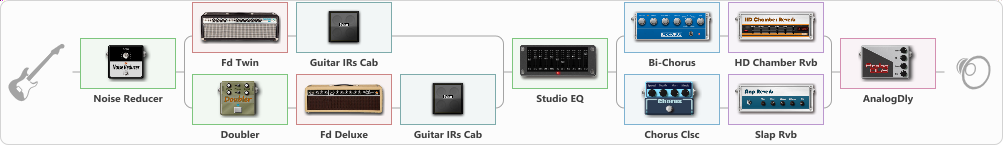Discussion in 'ToneLib-GFX presets' started by pepek, Jan 17, 2022.

Preset name: Chorus touch

Effects chain:Effect: "Noise Reducer" (Dynamics / Filter), active - "yes"
{
"Sens" = 28
"Mode" = Soft
}

Effect: "Splitter" (Dynamics / Filter)
{
"A-Bypass" = Off
"A-Pan" = -51
"A-Level" = 55
"B-Bypass" = Off
"B-Pan" = 52
"B-Level" = 63

'A' branch:
{

Effect: "Fd Twin" (Amp simulators), active - "yes"
{
"Gain" = 26
"Bass" = 64
"Middle" = 58
"Treble" = 62
"Presence" = 19
"Master" = 55
"Level (dB)" = 0
}

Effect: "Guitar IRs Cab" (Cabinets), active - "yes"
{
"Model" = Fender Twin Rev (2x12")
"Mic Position" = Middle
"Mic Distance" = Near
"Low Cut (Hz)" = 60
"Hi Cut (kHz)" = 20.0
"Mix" = 100
"Level (dB)" = 0
}
}
'B' branch:
{

Effect: "Doubler" (Dynamics / Filter), active - "yes"
{
"A-Dly" = 75
"A-Inv" = On
"A-Level" = 75
"B-Dly" = 68
"B-Inv" = On
"B-Level" = 75
}

Effect: "Fd Deluxe" (Amp simulators), active - "yes"
{
"Gain" = 27
"Bass" = 62
"Middle" = 61
"Treble" = 68
"Presence" = 36
"Master" = 62
"Level (dB)" = 0
}

Effect: "Guitar IRs Cab" (Cabinets), active - "yes"
{
"Model" = Vox AC30 (2x12")
"Mic Position" = Middle
"Mic Distance" = Near
"Low Cut (Hz)" = 60
"Hi Cut (kHz)" = 20.0
"Mix" = 100
"Level (dB)" = 0
}
}
}

Effect: "Studio EQ" (Dynamics / Filter), active - "yes"
{
"31 Hz" = -8
"62 Hz" = -3
"125 Hz" = 3
"250 Hz" = 3
"500 Hz" = 0
"1 kHz" = 0
"2 kHz" = -4
"4 kHz" = -4
"8 kHz" = -2
"16 kHz" = -4
"Level (dB)" = 7
}

Effect: "Splitter" (Dynamics / Filter)
{
"A-Bypass" = Off
"A-Pan" = 20
"A-Level" = 55
"B-Bypass" = Off
"B-Pan" = -20
"B-Level" = 55

'A' branch:
{

Effect: "Bi-Chorus" (Modulation / Sfx), active - "yes"
{
"Speed A" = 3.5
"Speed B" = 3.4
"Depth" = 43
"Reso" = 0
"Mode" = Parallel
"Mix" = 60
}

Effect: "HD Chamber Rvb" (Reverberation), active - "yes"
{
"Time" = 8.2
"PreLPF" = 79
"PreDelay" = 61
"HiDamp" = 21
"LoGain" = -2.1
"Mix" = 37
}
}
'B' branch:
{

Effect: "Chorus Clsc" (Modulation / Sfx), active - "yes"
{
"Speed" = 4.4
"Depth" = 46
"Center" = 1.0
"Mode" = Mono
}

Effect: "Slap Rvb" (Reverberation), active - "yes"
{
"Time" = 7.3
"PreDelay" = 0
"LoDamp" = 18
"HiDamp" = 40
"Mix" = 41
}
}
}

Effect: "AnalogDly" (Delay), active - "yes"
{
"Time" = 556
"Feedback" = 28
"Tone" = 65
"Mix" = 35
}

Note: You will need to download and install the ToneLib-GFX software to use the preset.